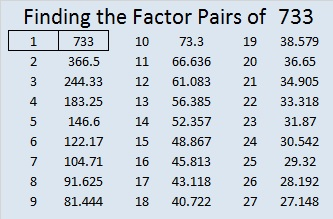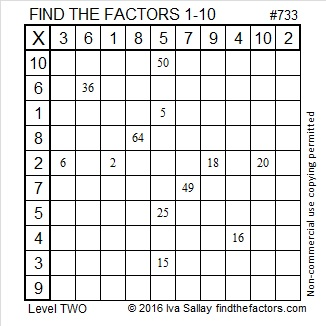# 733 and Level 2

• 733 is a prime number.
• Prime factorization: 733 is prime.
• The exponent of prime number 733 is 1. Adding 1 to that exponent we get (1 + 1) = 2. Therefore 733 has exactly 2 factors.
• Factors of 733: 1, 733
• Factor pairs: 733 = 1 x 733
• 733 has no square factors that allow its square root to be simplified. √733 ≈ 27.0739727.

How do we know that 733 is a prime number? If 733 were not a prime number, then it would be divisible by at least one prime number less than or equal to √733 ≈ 27.1. Since 733 cannot be divided evenly by 2, 3, 5, 7, 11, 13, 17, 19, or 23, we know that 733 is a prime number.

Here’s another way we know that 733 is a prime number: Since  its last two digits divided by 4 leave a remainder of 1, and 17² + 2² = 733 with 17 and 2 having no common prime factors, 733 will be prime unless it is divisible by a prime number Pythagorean hypotenuse less than or equal to √733 ≈ 27.1. Since 733 is not divisible by 5, 13, or 17, we know that 733 is a prime number.Give this Level 2 factoring puzzle a try:Print the puzzles or type the solution on this excel file: 10 Factors 2016-01-04

———————————————————

Here are some more facts about the number 733:

733 is prime, so 366 + 367 = 733 is the only way it can be written as the sum of consecutive numbers.

733 is the sum of the prime numbers from 17 to 79. I think there’s a good chance you know what all those prime numbers are.

733 is also the sum of the five prime numbers from 137  to 157. You might not know what the missing primes are, but here’s a hint: If you subtract 100 from any of those three primes, you will get a composite number, and there are only three odd composite numbers that don’t end in 5 between 37 and 57.

27² + 2² = 733 so 733 is the hypotenuse of the (primitive) Pythagorean triple 108-725-733 which is calculated from 2(27)(2), 27² – 2², 27² + 2².

Thus, 108² + 725² = 733².

733 is palindrome 292 in BASE 17; note that 2(17²) + 9(17) + 2(1) = 733.

From Wikipedia I learned two other interesting facts about the number 733:

• 727, 733, 739 are consecutive prime numbers whose average is 733.  That makes 733 the 13th balanced prime. It is exactly the same distance from the previous prime and the prime that follows it.
• 337, 373, 733 are three of only nine 3-digit permutable prime numbers. No matter their order, those exact 3 digits produce a prime number.

———————————————————Advertisements